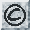The synthetic dataset "add10" uses a function suggested by Jerome Friedman in "Multivariate Adaptive Regression Splines", technical Report No. 102, November 1988, Laboratory for Computational Statistics, Department of Statistics, Stanford University.

The function is:
f(x1,...,x10) = 10*sin(pi*x1*x2) + 20*(x3-0.5)^2 + 10*x4 + 5*x5 + n, where n is zero mean unit variance Gaussian noise. The inputs x1...x10 are sampled independently from a uniform [0; 1] distribution.

## Dataset profile:

Origin: artificial

Usage: historical

Number of attributes: 11

Number of cases: 9,792

 Last Updated 21 October 1996 Comments and questions to: delve@cs.toronto.edu